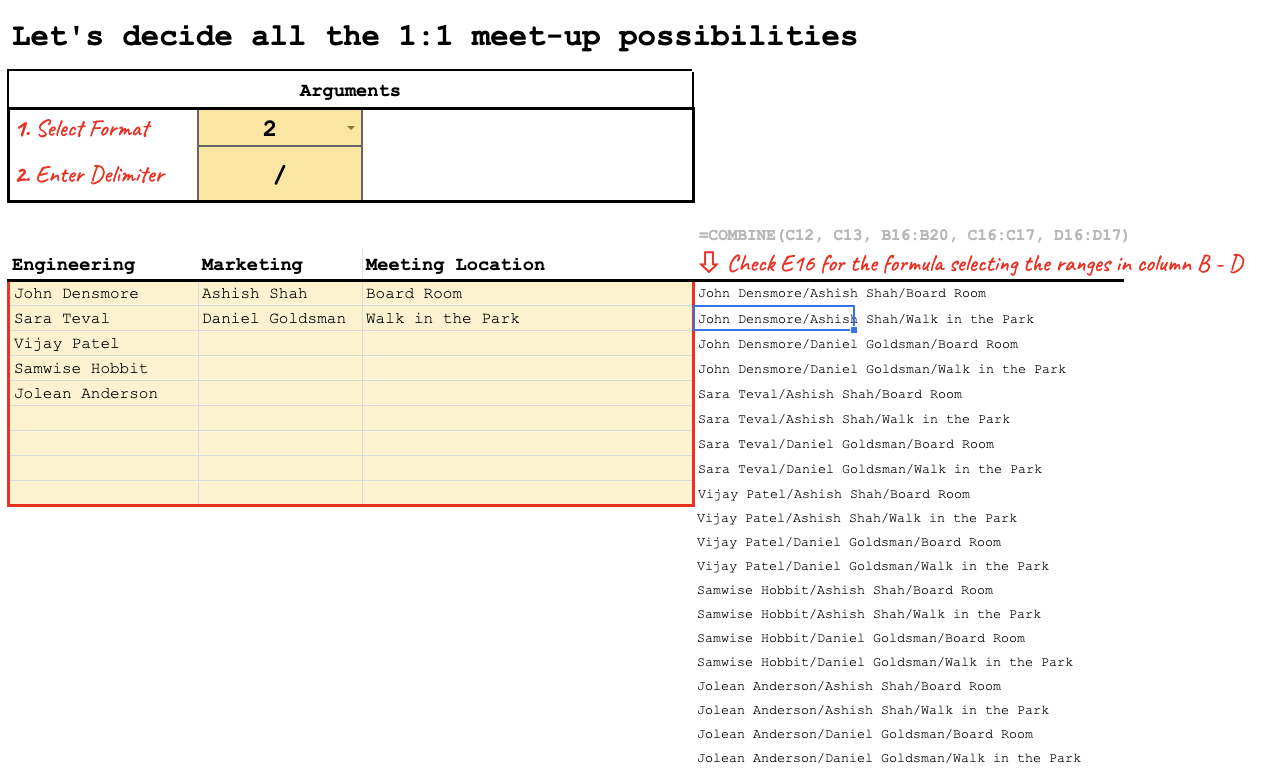# Get all combinations from sets in multiple lists in Google Sheets with COMBINE

Data GenerationLucas A. Browne Dias
Jul 7

The COMBINE function is a helpful tool that can make it dead simple to generate a list of combinations from other lists.

Whether you're looking to randomly select who's introduced to who, manage a menu of items and assign them to days, or solve a math problem for academic purposes, this function can help get you started.

### Function Signature

`=COMBINE(format, delimiter, ...arrays)`

### Argument Definitions

[number] format; required - how to generate combinations

• 1 - separate rows and columns
• 2 - separate rows
• 3 - single cell

[string] delimiter - also known as the separator for items in a given combination

[arrays] arrays - two or more ranges to combine

## Use the Cheat

Click the Code Box to Copy

Cheat copied to clipboard ✂️👍
``` /** * Creates all possible combinations from a group of data * * @param {int} format - How the combinations will be output. * Type 1 is in separate rows and columns; * * Type 2 is in separate rows; * * Type 3 is in a single cell * * @param {string} delimiter - The delimiter when joining values. Type 1: not used (must leave any value); Type 2: joins column values; Type 3: joins row values (no separation between column values). * @param {array} table - The data to create all combinations * * @return All possible combinations of the dataset. Eliminate duplicates by using UNIQUE. * * @customfunction */ function COMBINE(format = 1,delimiter = '', ...input){ let output_type = format; //variables let paramSize = []; let array = []; let inputSize = []; for (s=0; s < input.length; ++s){ //checks the size of each input argument inputSize.push(input[s].length) }; let arraySize = inputSize.reduce( function (p, v) { return ( p > v ? p : v ) } ); //sets the size of the unified input for (k=0; k < arraySize; k++){array.push([])}; for(a=0; a < input.length; a++){ //creates the unified input out of all arguments if(Array.isArray(input[a])){ for (b=0; b < input[a].length; b++){ let length = input[a].length; paramSize.push(length); for (s=0;s<arraySize;s++){ array[s].push( s >= length ? 0 : input[a][s][b]); } } } else { //single value option paramSize.push(1); array.push(input[a]); for (s=1;s<arraySize;s++) { array[s].push(0); } } } let iterations = 1; //number of the column iterated for(i=0; i < paramSize.length ;i++){ //multiplies all paramSize values to find how many combinations there will be iterations = iterations * paramSize[i]; } var combinations = []; for (c=0 ; c < iterations; c++) { combinations[c]=[]; } //creates a array for each row of combinations to be inputed var rounds = 1; //times all column values will go around var repititions = iterations; //times each value will repeat itself before going to next value for(p=0; p<paramSize.length; p++){ repititions = repititions/paramSize[p]; //repetitions decrease for each column for(n=0;n<rounds;++n){ for(c=0; c < paramSize[p]; c++) { for(r=0; r < repititions; r++) { row = (n * paramSize[p] * repititions) + c * repititions + r; combinations[row].push(array[c][p]); } } } rounds = rounds*paramSize[p]; //number of rounds increase for each column }; let output = []; switch (output_type){ case 1: output = combinations; break; case 2: for(r=0; r < combinations.length; r++) { var outputRow = ''; for(c=0; c < combinations.length; c++){ outputRow += String(combinations[r][c]) + delimiter; }; output.push([outputRow.slice(0,outputRow.length - delimiter.length)]) } ; break; case 3: let arr = [] for(r=0; r < combinations.length; r++) { var rowString = ''; const clen = combinations.length; for(c=0; c < clen; c++) { rowString += String(combinations[r][c]); if (c < clen - 1) { rowString += delimiter; } } arr.push(rowString); } output.push(arr.join(",")); break; default: output.push(['#Invalid Type!']) break; } return output; } ```